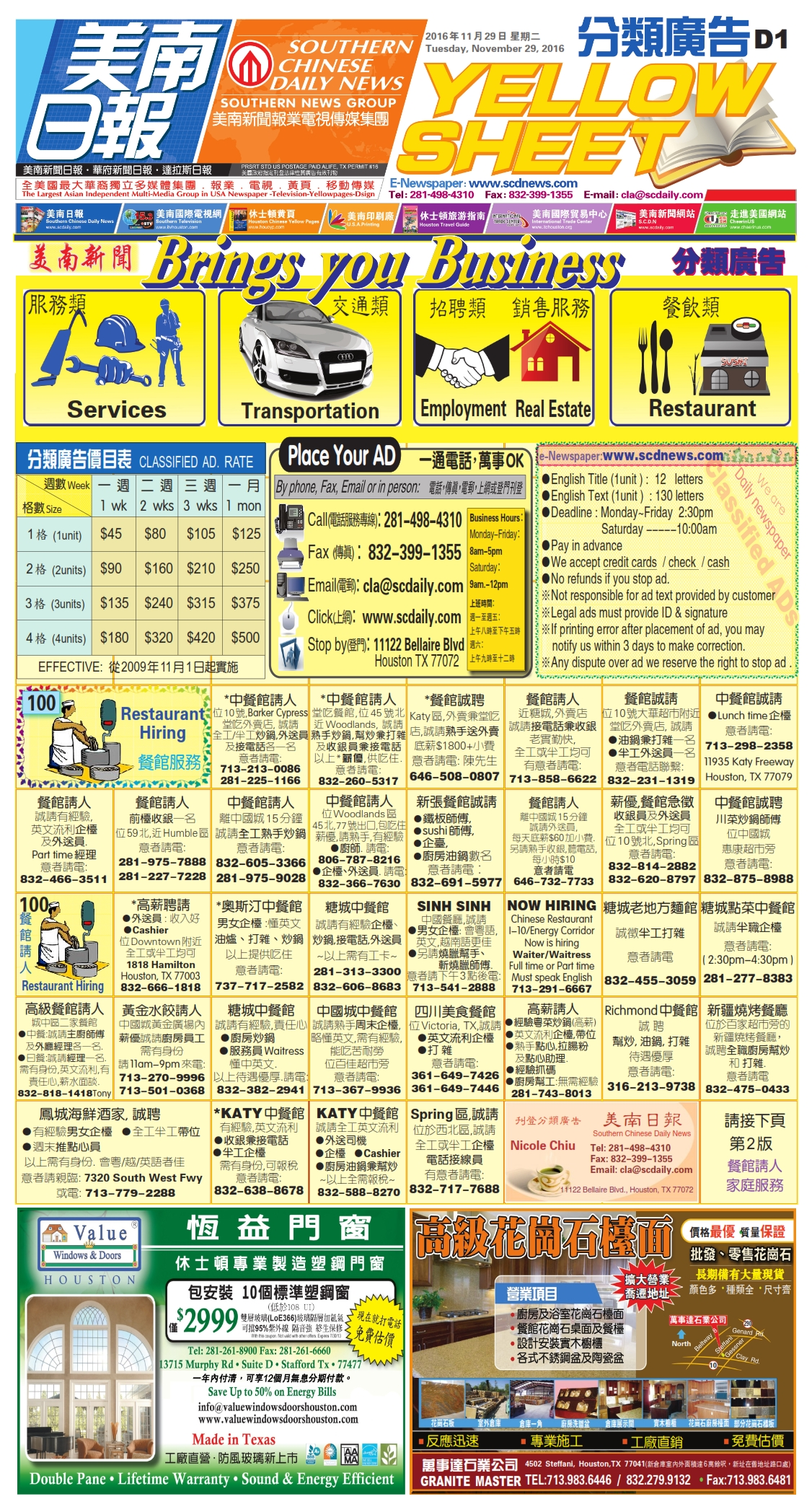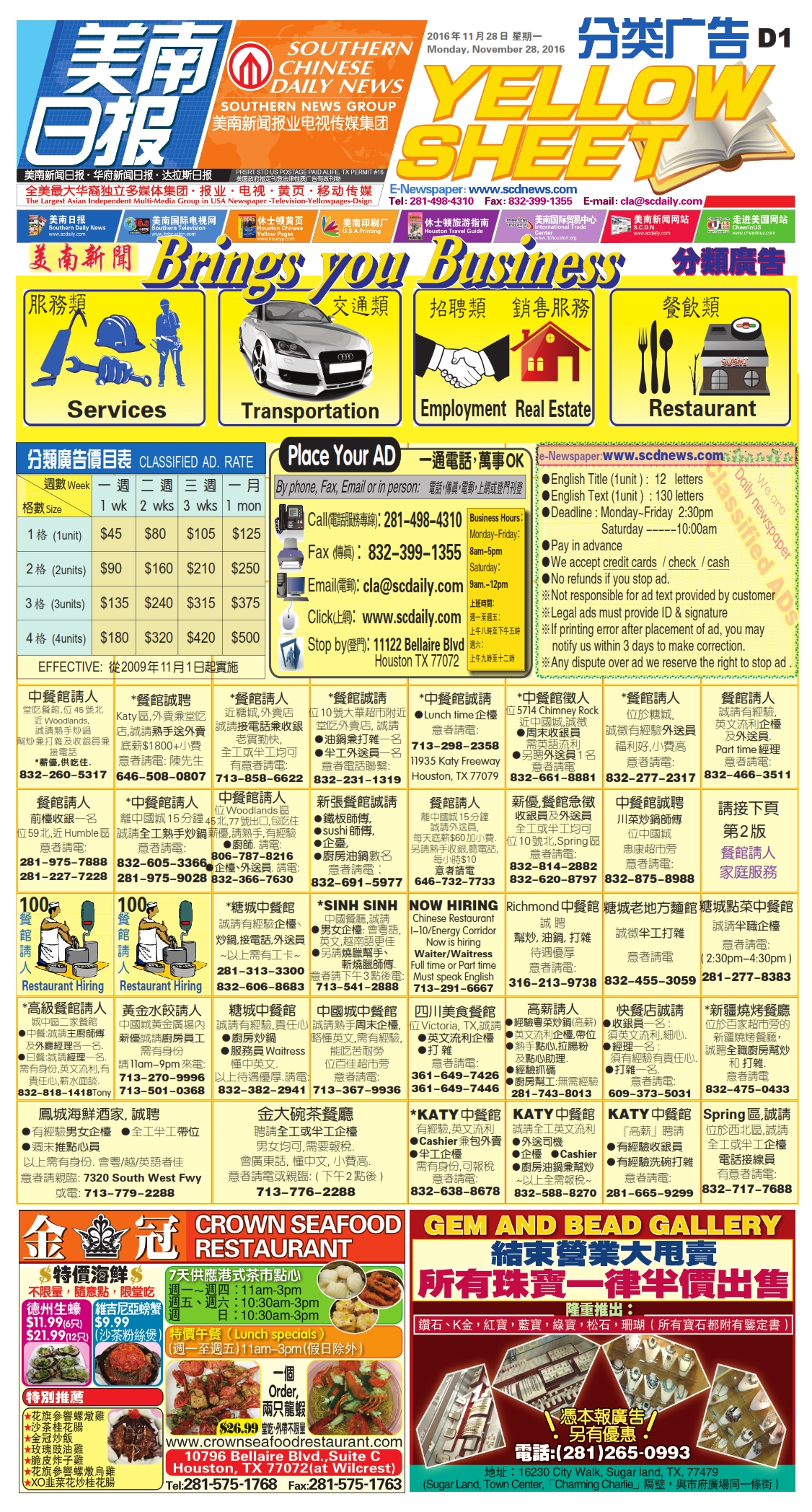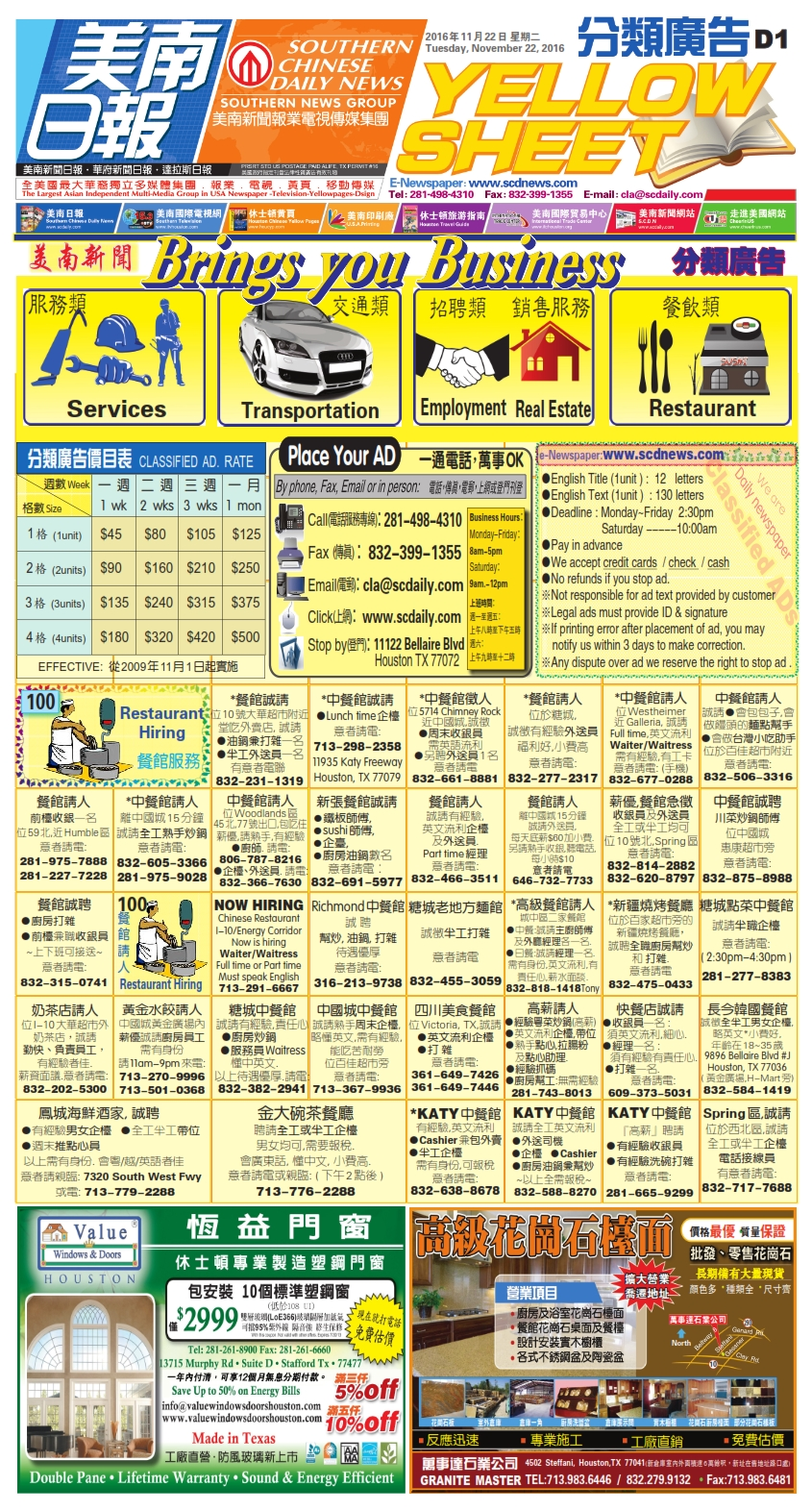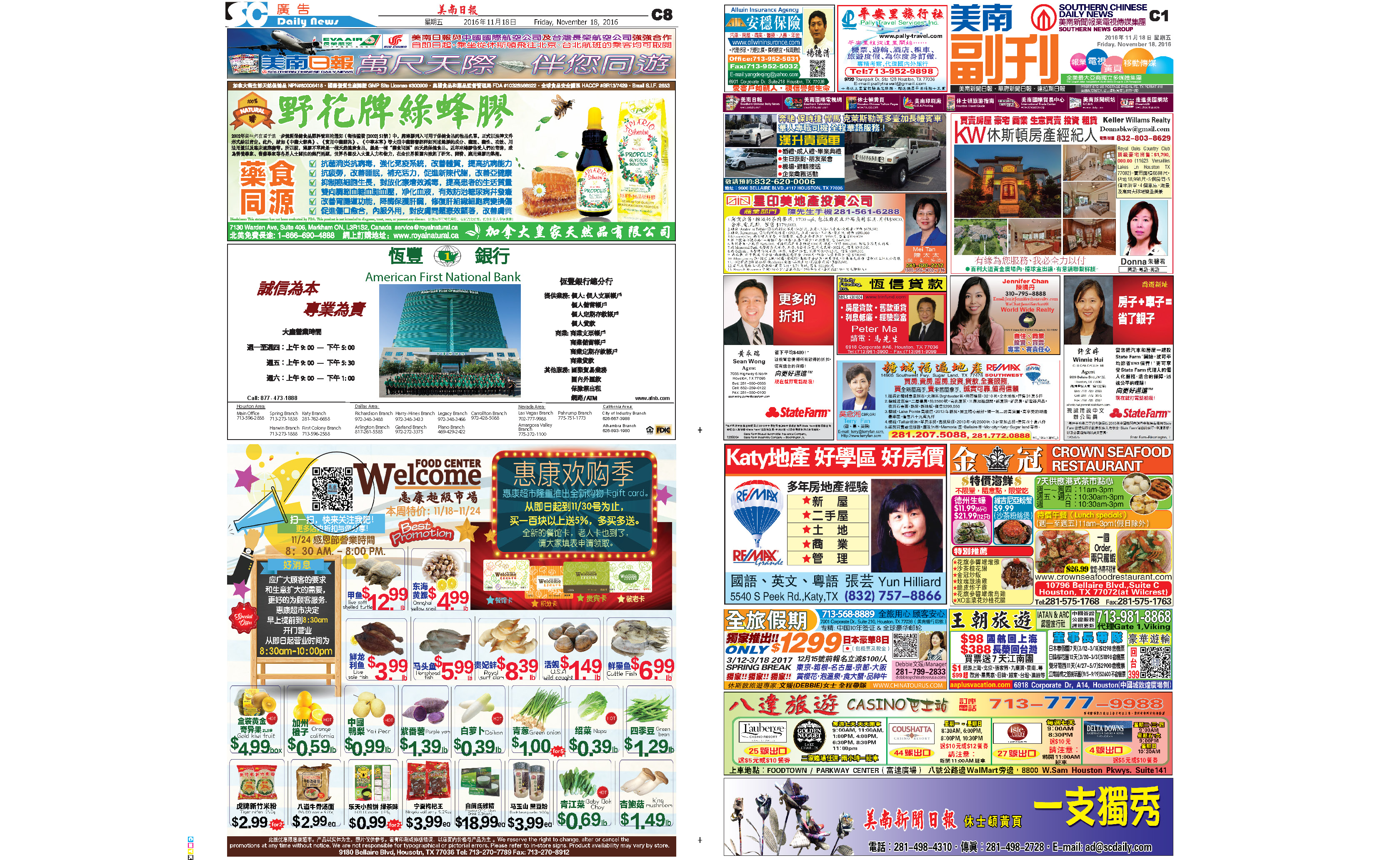161129 Epaper

 A Section B SectionC Section D Section161128 Epaper

 A Section B SectionC Section D Section161127 Epaper

 A Section B SectionC Section D Section161126 Epaper

 A Section B SectionC Section D Section161125 Epaper

 A Section B SectionC Section D Section161124 Epaper

 A Section B SectionC Section D Section161123 Epaper

 A Section B SectionC Section D Section161122 Epaper

 A Section B SectionC Section D Section161120 Epaper

 A Section B SectionC Section D Section161121 Epaper

 A Section B SectionC Section D Section161118 Epaper

 A Section B SectionC Section D Section